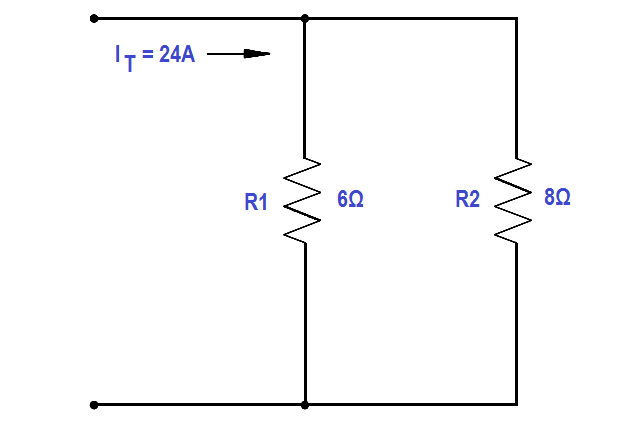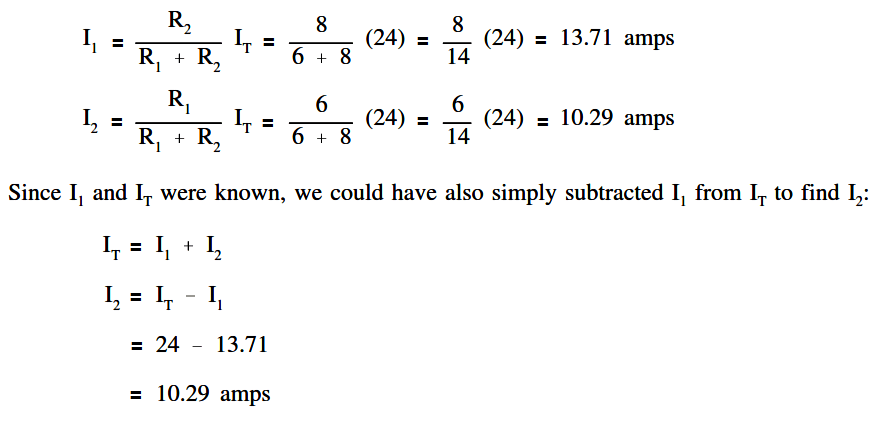# Current Division

Sometimes it is necessary to find the individual branch currents in a parallel circuit when only resistance and total current are known. When only two branches are involved, the current in one branch will be some fraction of IT . The resistance in each circuit can be used to divide the total current into fractional currents in each branch. This process is known as current division.Note that the equation for each branch current has the opposite R in the numerator. This is because each branch current is inversely proportional to the branch resistance.

Example:

Find branch current for I 1 and I 2 for the circuit shown in Figure 30.Figure 30 Current Division Example Circuit

Solution: